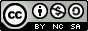## Pop. Calc. Solutions

Solution to Problem Set 1

1) 102000

r * N   so 7.5 * 12000 = 9000 growth.    Add growth to start population so 90000 + 12000

2) 21218

r * N so after 1 year growth = 0.03 * 20000 = 600 so the population is 20600

after two years 0.03 * 20600  = 618  so the population is 21218

3) 13860

r * N  so   -0.01 * 14000 = -140   so population equals 14000 + (-140)

4) 293     292.8, but you can't have 0.8 fleas, so 293

r*N    0.1 * 200 = 20 so 220   then week 2     0.1*220=22  so 242      then week 3     0.1*242=24.2   so 266.2

then week 4    0.1*266.2 = 26.6    so   292.8

Solutions to Problem Set 2

1) birth rate = 0.048 ;     death rate = 0.04

birth rate= 12000/250000    and   death rate = 10000/250000

2) 0.008   ;      growing

growth rate = birth rate - death rate  so 0.048 - 0.04

3) birth rate = 0.047 and death rate = 0.048

birth rate = 109000/2300000     and      death rate = 111000/2300000

4) growth rate =  -0.001   ;   shrinking

growth rate = birth rate - death rate  so  0.047 - 0.048

5) growth rate = 0.01

birth rate = 30/1500   and death rate = 15/1500 so

birth rate = 0.02 and death rate = 0.01

growth rate = birth rate - death rate

6) population = 227700

population growth = r * N  so    -0.001 * 2300000  = -2300

Population equals initial population + growth   so     2300000 + (-2300)

7) Population after 3 years = 256047

after one year 0.008 * 250000 = 2000 growth  so population after 1 year is 252000

after two years 0.008 * 252000 = 2016 growth so population after 2 years is 254016

after three years 0.008 * 254016 = 2032 growth so population after 3 years is 256048Brett Dooley Website Maintained since 2011 Last Updated July 2019